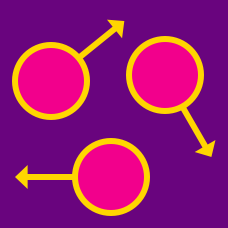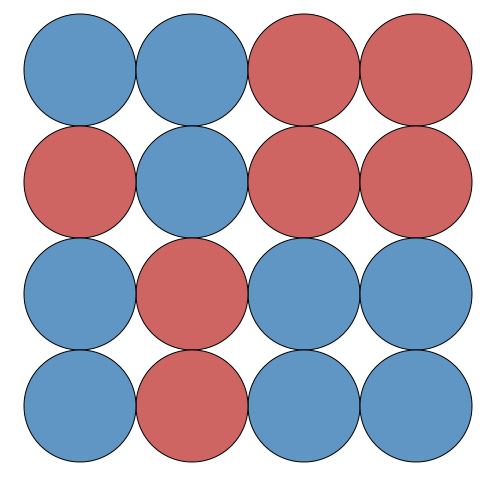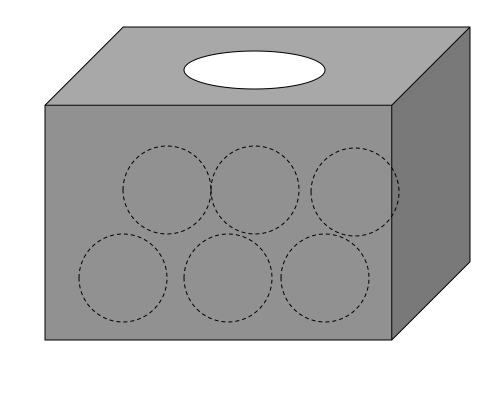Classical Mechanics

# Microstates and macrostatesThere are counters, which are blue on one side and red on the other. If the macroscopic description of our state of interest is $8$ red on the $4\times 4$ checkerboard, how many corresponding micro-states are there?

A coin is tossed $8$ times, and it is noted that the number of heads is $3.$ How many sequences of coin flips could have led to this result?

There are $15$ pigeon-holes for $2$ pigeons. If at most one pigeon can fit inside each hole, how many distinct arrangements are there for the $2$ pigeons to fit inside the $15$ holes?

Assume that we can distinguish each pigeon from the others.There are six distinct boxes, each of which contains a single ball. If there are three red balls and three blue balls, how many distinct arrangements are possible?

There are $5$ pigeon-holes for $5$ pigeons. Three of the pigeons are white, and the other two are grey. If each hole can hold at most a single pigeon, how many micro-states are there for the $5$ pigeons to fit into the 5 hole?

Assume that we can distinguish each pigeon by their color, but cannot distinguish two pigeons of the same color.

×